# Chapter 10: Light Reflection and Refraction

Course Content
Science (NCERT Solutions)
0/16
Science (MCQ)
0/16
Science (Video Lessons in Assamese)
0/7
Science (Test Yourself)
0/1
Maths (Video Lessons in Assamese)
Maths (MCQ)
0/8
Maths (Test Yourself)
0/3
Maths (1 mark question)
0/4
Class 10 : A Complete Package
Questions Page: 1681. Define the principal focus of a concave mirror.

Light rays that are parallel to the principal axis of a concave mirror converge at a specific point on its principal axis after reflecting from the mirror. This point is called the principal focus of the concave mirror.

2. The radius of curvature of a spherical mirror is 20 cm. What is its focal length?

Radius of curvature (R) = 20 cm

Radius of curvature of the spherical mirror = 2 × Focal length (f)

R = 2f

f= R/2 = 20 / 2 = 10

Therefore, the focal length of the spherical mirror is 10 cm.

3. Name the mirror that can give an erect and enlarged image of an object.

The mirror that can give an erect and enlarged image of an object is a Concave Mirror.

4. Why do we prefer a convex mirror as a rear-view mirror in vehicles?

A convex mirror is preferred as a rear-view mirror in cars and vehicles as it gives a wider field of view, which helps the driver see most of the traffic behind him. Convex mirrors always form an erect, virtual, and diminished image of the objects placed in front of it.

Page No: 171

1. Find the focal length of a convex mirror whose radius of curvature is 32 cm.

Radius of curvature (R) = 32 cm

Radius of curvature = 2 × Focal length (f)

R= 2f

f = R/2 = 32/2 = 16

Therefore, the focal length of the given convex mirror is 16 cm.

2. A concave mirror produces three times magnified (enlarged) real image of an object placed at 10 cm in front of it. Where is the image located?

Magnification produced by a spherical mirror: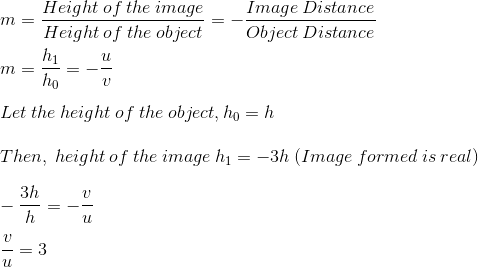Object distance (u) = – 10 cm

v = 3 × (- 10) = – 30 cm

Therefore, the negative sign indicates that an inverted image is formed in front of the given concave mirror at a distance of 30 cm.

Page No: 176

1. A ray of light travelling in air enters obliquely into water. Does the light ray bend towards the normal or away from the normal? Why?

The light ray bends towards the normal. When a light ray enters from an optically rarer medium (which has a low refractive index) to an optically denser medium (which has a high refractive index), its speed slows down and bends towards the normal. As water is optically denser than air, a ray of light entering from air into water will bend towards the normal.

2. Light enters from air to glass, having a refractive index 1.50. What is the speed of light in the glass? The speed of light in vacuum is 3 x 108 ms-1.

Refractive index of a medium (nm) = Speed of light in vacuum/Speed of light in the medium

Speed of light in vacuum (c) = 3 × 108 m/s

Refractive index of glass (ng) = 1.50

Speed of light in the glass (v) = Speed of light in vacuum/ Refractive index of glass

= c/ng

=3 × 108/1.50 = 2x 108 ms-1.

3. Find out, from the table, the medium having the highest optical density. Also, find the medium with the lowest optical density.

 Materialmedium Refractive index Material medium Refractiveindex Air 1.0003 Canada Balsam 1.53 Ice 1.31 – – Water 1.33 Rock salt 1.54 Alcohol 1.36 – – Kerosene 1.44 Carbon disulphide 1.63 Fusedquartz 1.46 Denseflint glass 1.65 Turpentine oil 1.47 Ruby 1.71 Benzene 1.50 Sapphire 1.77 Crownglass 1.52 Diamond 2.42

Lowest optical density = Air

Highest optical density = Diamond

The optical density of a medium is directly related to its refractive index. A medium with the highest refractive index will have the highest optical density and vice-versa.

It can be observed from the table that air and diamond, respectively have the lowest and highest refractive index. Hence, air has the lowest optical density and diamond has the highest optical density.

4. You are given kerosene, turpentine and water. In which of these does the light travel fastest? Use the information given in the table.

 Materialmedium Refractive index Material medium Refractiveindex Air 1.0003 Canada Balsam 1.53 Ice 1.31 – – Water 1.33 Rock salt 1.54 Alcohol 1.36 – – Kerosene 1.44 Carbon disulphide 1.63 Fusedquartz 1.46 Denseflint glass 1.65 Turpentine oil 1.47 Ruby 1.71 Benzene 1.50 Sapphire 1.77 Crownglass 1.52 Diamond 2.42

Light travels faster in water as compared to kerosene & turpentine, as the refractive index of water is lower than that of kerosene and turpentine. The speed of light is inversely proportional to the refractive index.

5. The refractive index of diamond is 2.42. What is the meaning of this statement?

A diamond has a refractive index of 2.42, which means that the speed of light in a diamond will reduce by a factor of 2.42 as compared to its speed in the air.

In other words, the speed of light in a diamond is 1/2.42 times the speed of light in a vacuum.

Page No: 184

1. Define 1 dioptre of power of a lens.

Dioptre is the SI unit of power of lens is denoted by the letter D. 1 dioptre can be defined as the power of a lens of focal length 1 metre.

2. A convex lens forms a real and inverted image of a needle at a distance of 50 cm from it. Where is the needle placed in front of the convex lens if the image is equal to the size of the object? Also, find the power of the lens.

The position of the image should be at 2F since the image is real and the same size.

It is given that the image of the needle is formed at a distance of 50 cm from the convex lens. Therefore, the needle is placed in front of the lens at a distance of 50 cm.

Object distance (u) = – 50 cm

Image distance, (v) = 50 cm

Focal length = f

According to the lens formula,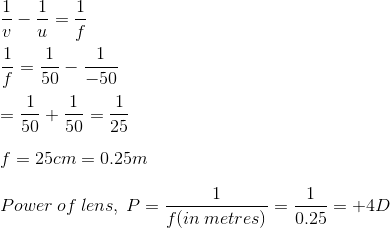3. Find the power of a concave lens of focal length 2 m.

The focal length of the concave lens (f) = 2 m

Power of lens (P) = 1/f = 1/ (-2) = -0.5D

Page No: 185

Exercise

1. Which one of the following materials cannot be used to make a lens?

(a) Water

(b) Glass

(c) Plastic

(d) Clay

(d) Clay cannot be used to make a lens because if the lens is made up of clay, the light rays cannot pass through it

2. The image formed by a concave mirror is observed to be virtual, erect and larger than the object. Where should be the position of the object?

(a) Between the principal focus and the centre of curvature

(b) At the centre of curvature

(c) Beyond the centre of curvature

(d) Between the pole of the mirror and its principal focus.

(d) The position of the object should be between the pole of the mirror and its principal focus.

3. Where should an object be placed in front of a convex lens to get a real image of the size of the object?

(a) At the principal focus of the lens

(b) At twice the focal length

(c) At infinity

(d) Between the optical centre of the lens and its principal focus.

(b) The object should be placed at twice the focal length

4. A spherical mirror and a thin spherical lens have a focal length of -15 cm. The mirror and the lens are likely to be

(a) both concave

(b) both convex

(c) the mirror is concave, and the lens is convex

(d) the mirror is convex, but the lens is concave

(a) Both are likely to be concave.

Page No: 186

5. No matter how far you stand from a mirror, your image appears erect. The mirror is likely to be

(a) plane

(b) concave

(c) convex

(d) either plane or convex

(d) The mirrors are likely to be either plane or convex

6. Which of the following lenses would you prefer to use while reading small letters found in a dictionary?

(a) A convex lens of focal length 50 cm

(b) A concave lens of focal length 50 cm

(c) A convex lens of focal length 5 cm

(d) A concave lens of focal length 5 cm

(c) A convex lens of focal length 5 cm can be used while reading small letters found in a dictionary

7. We wish to obtain an erect image of an object, using a concave mirror of focal length 15 cm. What should be the range of distance of the object from the mirror? What is the nature of the image? Is the image larger or smaller than the object? Draw a ray diagram to show the image formation in this case.

Range of the distance of the object = 0 to 15 cm from the pole of the mirror.

Nature of the image = virtual, erect, and larger than the object.

8. Name the type of mirror used in the following situations.

(b) Side/rear-view mirror of a vehicle

(c) Solar furnace

(a) Concave Mirror: Concave mirrors can produce a powerful parallel beam of light when the light source is placed at their principal focus.

(b) Convex Mirror: Because of its largest field of view.

(c) Concave Mirror: Because it concentrates the parallel rays of the sun at a principal focus.

9. One-half of a convex lens is covered with black paper. Will this lens produce a complete image of the object? Verify your answer experimentally. Explain your observations.

Yes, it will produce a complete image of the object, as shown in the figure. This can be verified experimentally by observing the image of a distant object, like a tree on a screen, when the lower half of the lens is covered with black paper. However, the intensity or brightness of the image will reduce.

10. An object 5 cm in length is held 25 cm away from a converging lens of focal length 10 cm. Draw the ray diagram and find the position, size and nature of the image formed.

Height of the object, h0 = 5 cm

Distance of the object from converging lens, u = -25 cm

Focal length of a converging lens, f = 10 cm

Using the lens formula,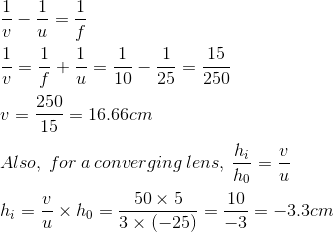Thus, the image is inverted and formed at a distance of 16.7 cm behind the lens and measures 3.3 cm. The ray diagram is shown below.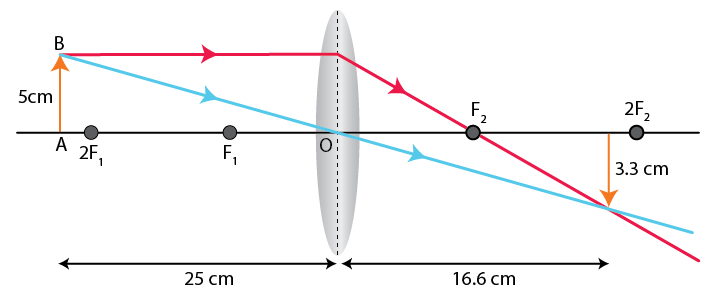11. A concave lens of focal length 15 cm forms an image 10 cm from the lens. How far is the object placed from the lens? Draw the ray diagram.

Focal length of concave lens (OF1), f = – 15 cm

Image distance, v= – 10 cm

According to the lens formula,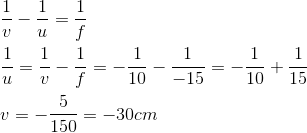The negative value of u indicates that the object is placed 30 cm in front of the lens. This is shown in the following ray diagram.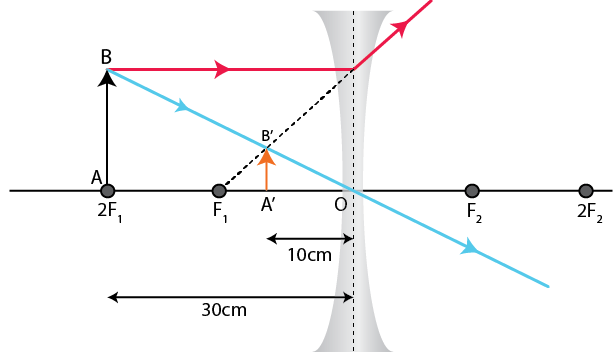12. An object is placed at a distance of 10 cm from a convex mirror of focal length 15 cm. Find the position and nature of the image.

Focal length of convex mirror (f) = +15 cm

Object distance (u) = – 10 cm

According to the mirror formula,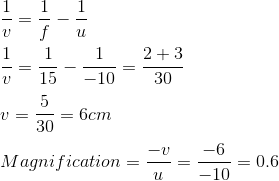The image is located at a distance of 6 cm from the mirror on the other side of the mirror.

The positive and a value of less than 1 magnification indicates that the image formed is virtual, erect, and diminished.

13. The magnification produced by a plane mirror is +1. What does this mean?

The positive sign means an image formed by a plane mirror is virtual and erect. Since the magnification is 1, it means that the size of the image is equal to the size of the object.

14. An object 5 cm is placed at a distance of 20 cm in front of a convex mirror of radius of curvature 30 cm. Find the position, nature and size of the image.

Object distance (u) = – 20 cm

Object height (h) = 5 cm

Radius of curvature (R) = 30 cm

Radius of curvature = 2 × Focal length

R = 2f

f = 15 cm

According to the mirror formula,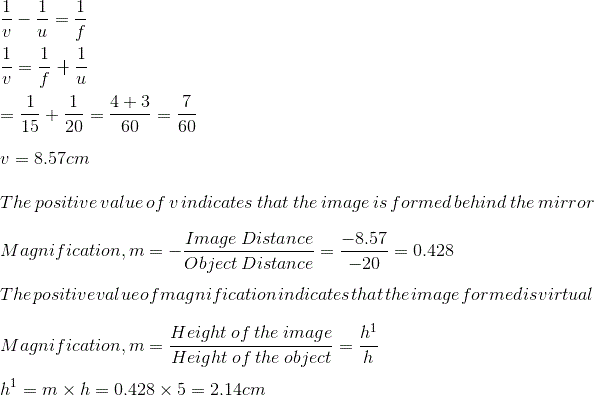The positive value of image height indicates that the image formed is erect.

Hence, the image formed is erect, virtual, and smaller in size.

15. An object of size 7.0 cm is placed at 27 cm in front of a concave mirror of focal length 18 cm. At what distance from the mirror should a screen be placed so that a sharply focused image can be obtained? Find the size and nature of the image.

Object distance (u) = – 27 cm

Object height (h) = 7 cm

Focal length (f) = – 18 cm

According to the mirror formula,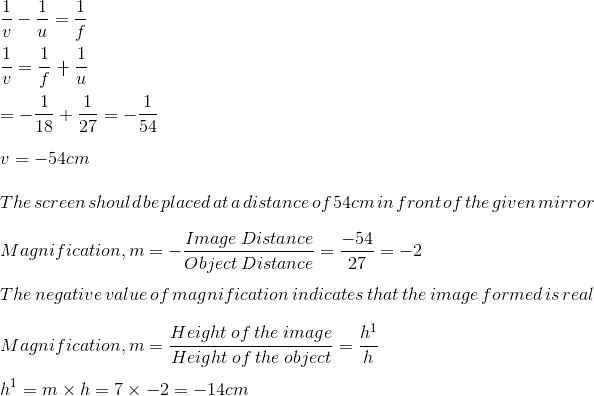The negative value of image height indicates that the image formed is inverted.

16. Find the focal length of a lens of power -2.0 D. What type of lens is this?

Power of lens (P) = 1/f

P = -2D

f = -1/2 = -0.5 m

A concave lens has a negative focal length. Therefore, it is a concave lens.

17. A doctor has prescribed a corrective lens of power +1.5 D. Find the focal length of the lens. Is the prescribed lens diverging or converging?